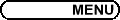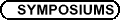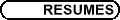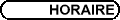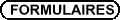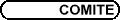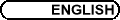Classical Analysis in honour of David Borwein's 80th Birthday / Analyse classique en l'honneur du 80eme anniversaire de David Borwein(Org: Jonathan Borwein, Dalhousie University, and/et Mike Overton, New York University) HEINZ BAUSCHKE, University of Guelph Asymptotic behaviour of the composition of two resolvents Consider the problem of finding a point in the intersection of two nonempty closed convex sets in a Hilbert space. The method of alternating projections is an iterative attempt to solve this problem. If the intersection of the two sets is nonempty, then-this is a classical result due to Bregman-the sequence generated converges weakly to a common point; otherwise, the sequence either tends to infinity in norm or it possesses two cluster points realizing the gap between the two sets. In this talk, I will discuss recent extensions of this result concerning the asymptotic behaviour of resolvents and Bregman proximal maps. Based on joint work with Patrick Combettes (Paris 6), Dominikus Noll (Toulouse), and Simeon Reich (Technion). GRAHAME BENNETT, Indiana University, Bloomington, IN  47405, USA Summability for those without We consider several attractive and easy-to-state problems. These have no apparent connection with Summability Theory, yet their solutions are all centered upon one of David Borwein's favorite topics: Hausdorff matrices. PETER BORWEIN, Mathematics Department, Simon Fraser University The Mahler Measure of Polynomials with Odd Coefficients We resolve an old conjecture of Schinzel and Zassenhaus for the class of polynomials with no cyclotomic factors whose coefficients are all odd. More generally, we solve the problems of Lehmer for irreducible polynomials in the above class by showing that the Mahler measure (the L0 norm) of such polynomials is bounded away from 1. PETER CASS, University of Western Ontario, London, Ontario A Retrospective on David Borwein's contribution to mathematical research A retrospective on David Borwein's contribution to mathematical research. MICHAEL OVERTON, Courant Institute of Mathematical Sciences, New York University, 251 Mercer St., New York, NY  10012, USA Optimal Stability and Eigenvalue Multiplicity We consider the problem of minimizing over an affine set of square matrices the maximum of the real parts of the eigenvalues. Such problems are prototypical in robust control and stability analysis. Under nondegeneracy conditions, we show that the multiplicities of the active eigenvalues at a critical matrix remain unchanged under small perturbations of the problem. Furthermore, each distinct active eigenvalue corresponds to a single Jordan block. This behavior is crucial for optimality conditions and numerical methods. Our techniques blend nonsmooth optimization and matrix analysis. This is joint work with James V. Burke (U. Wash.) and Adrian S. Lewis (SFU). BRUCE SHAWYER, Memorial University of Newfoundland Tribute and a Plethora of Remarkable Concurrences This talk is in two parts: An appreciation of David Borwein as a colleague, problem solver, supervisor and person. A Plethora of Remarkable Concurrences, dedicated to David Borwein on his 80th birthday. There are several examples of concurrence in classical triangle geometry. For example, the medians, the altitudes, the angle bisectors and the perpendicular bisectors of the sides. Here we show that there are a plethora of concurrences with some remarkable properties. BRUCE WATSON, Memorial University, St. John's, NL Discrete Power Series Methods In 1989 and 1990 papers, Maddox and Armitage discretized the well-known Cesàro and Abel methods. The author extends the definitions to discrete power series and weighted mean methods and gives some abelian and tauberian results. JIM ZHU, Western Michigan University A variational proof of Birkhoff's theorem on doubly stochastic matrices In this talk I will give a short and self-contained variational proof of Birkhoff's theorem asserting that the extreme points of the convex set of doubly stochastic matrices are the permutation matrices.